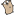## Go by Example 中文：切片

 Slice 是 Go 中一个关键的数据类型，是一个比数组更加强大的序列接口```package main ``` ```import "fmt" ``` ```func main() { ``` 不像数组，slice 的类型仅由它所包含的元素决定（不像数组中还需要元素的个数）。要创建一个长度非零的空slice，需要使用内建的方法 `make`。这里我们创建了一个长度为3的 `string` 类型 slice（初始化为零值）。 ``` s := make([]string, 3) fmt.Println("emp:", s) ``` 我们可以和数组一样设置和得到值 ``` s = "a" s = "b" s = "c" fmt.Println("set:", s) fmt.Println("get:", s) ``` 如你所料，`len` 返回 slice 的长度 ``` fmt.Println("len:", len(s)) ``` 作为基本操作的补充，slice 支持比数组更多的操作。其中一个是内建的 `append`，它返回一个包含了一个或者多个新值的 slice。注意我们接受返回由 append返回的新的 slice 值。 ``` s = append(s, "d") s = append(s, "e", "f") fmt.Println("apd:", s) ``` Slice 也可以被 `copy`。这里我们创建一个空的和 `s` 有相同长度的 slice `c`，并且将 `s` 复制给 `c`。 ``` c := make([]string, len(s)) copy(c, s) fmt.Println("cpy:", c) ``` Slice 支持通过 `slice[low:high]` 语法进行“切片”操作。例如，这里得到一个包含元素 `s`, `s`,`s` 的 slice。 ``` l := s[2:5] fmt.Println("sl1:", l) ``` 这个 slice 从 `s` 到（但是包含）`s`。 ``` l = s[:5] fmt.Println("sl2:", l) ``` 这个 slice 从（包含）`s` 到 slice 的后一个值。 ``` l = s[2:] fmt.Println("sl3:", l) ``` 我们可以在一行代码中声明并初始化一个 slice 变量。 ``` t := []string{"g", "h", "i"} fmt.Println("dcl:", t) ``` Slice 可以组成多维数据结构。内部的 slice 长度可以不同，这和多位数组不同。 ``` twoD := make([][]int, 3) for i := 0; i < 3; i++ { innerLen := i + 1 twoD[i] = make([]int, innerLen) for j := 0; j < innerLen; j++ { twoD[i][j] = i + j } } fmt.Println("2d: ", twoD) } ```
 注意，slice 和数组不同，虽然它们通过 `fmt.Println` 输出差不多。 ```\$ go run slices.go emp: [ ] set: [a b c] get: c len: 3 apd: [a b c d e f] cpy: [a b c d e f] sl1: [c d e] sl2: [a b c d e] sl3: [c d e f] dcl: [g h i] 2d: [ [1 2] [2 3 4]] ``` 看看这个由 Go 团队撰写的一篇很棒的博文，获得更多关于 Go 中 slice 的设计和实现细节。 现在，我们已经看过了数组和 slice，接下来我们将看看 Go 中的另一个关键的内建数据类型：map。